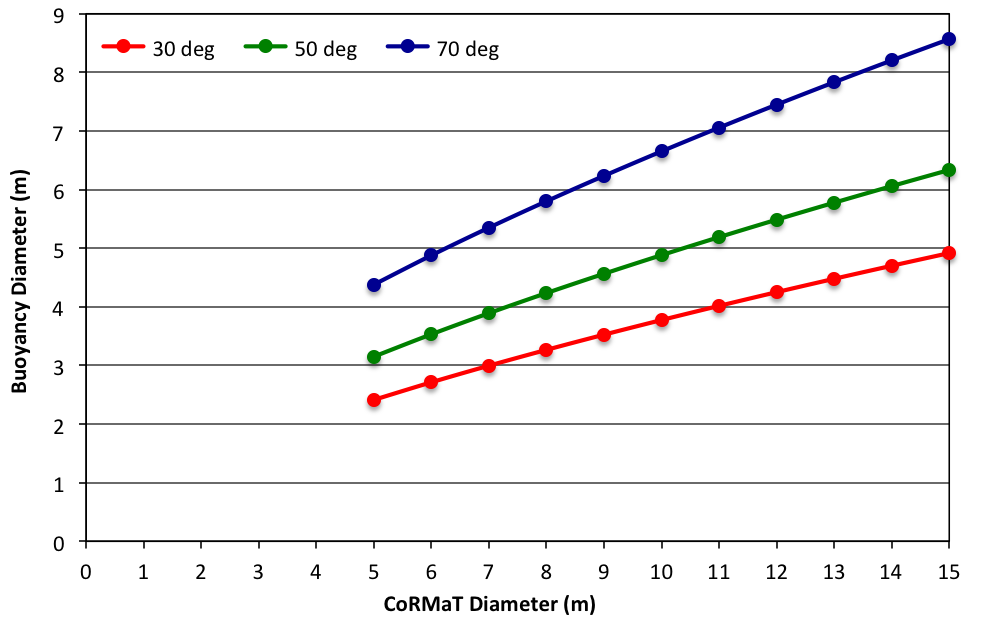BUOY

## Mooring Angles

The chart below shows the relationship between the CoRMaT turbine diameter and the buoy size, in order to maintain various mooring angles (between the seabed and the mooring line). Note that the Buoyancy Diameter in this case is for a spherical buoy. For a 10 m rotor diameter turbine, in order to maintain a 50 degree mooring angle in a 3.0 m/s tidal current (which was the design case for this Project) it was found that a 5 m diameter spherical buoy is required. Large size sub-surface buoyancy is more typically cylindrical rather than spherical, therefore a 4 m diameter and 5 m high cylindrical buoy would be required.In order to calculate the mooring angle, the drag forces on both the turbine and the buoyancy were calculated, as well as the buoyancy force. The Turbine Drag section explains how the drag coefficient of the turbine was derived for use in these calculations.